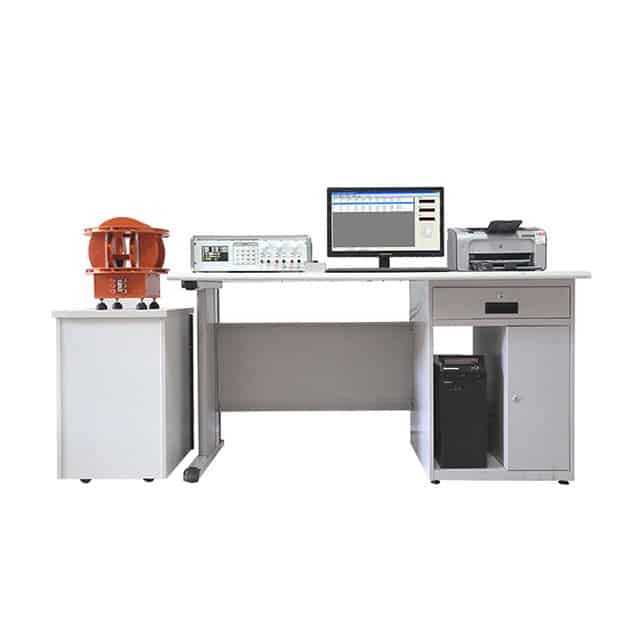#### products categories

• Tel : 18030236818
• Fax : +86-592 5237901
• Email : dexing@china-dexing.com

# How to measure magnetic declination

Measuring principle of magnetic declination:

1. The magnetic steel that has been magnetized is measured with Helmholtz coil, and the magnetic torque Mx, My and Mz in three directions are obtained (the actual measurement is the magnetic flux in three directions, which is multiplied by the coil constant K). Each quantity is corresponding to the X, Y and Z quantities in the rectangular coordinates, and the vector is synthesized, which is the actual magnetizing direction. If we want to find the included Angle A with the main magnetizing direction (such as X direction), we only need to find the tangent of the included Angle A. The tangent is: the sum of the square root of My and Mz, and then divide by Mx. The corresponding included Angle can be calculated by looking up the table or using a calculator.
II. The 3D Helmholtz coil and three-channel magnetometer are used to directly measure the magnetic moment Mx, My and Mz in three directions, and the vector and vector synthesis in three directions can be directly displayed with the automatic control of single-chip microcomputer and the calculation software. The deflection Angle with the magnetizing direction can be directly selected, and it can be compared with the pre-value Angle to determine whether it conforms to the control range of the magnetizing deflection Angle.
Three samples, transmission mechanism and fluxmeter choice: a sample organization in order to meet a person operating, using and orbit control, track length should be more than one meter, the concrete operation process is: the samples will be tested installed on a fixed card slot (consistent absolute guarantee that the magnetization direction and the direction of the main coil), launch the test, the sample delivered to coil center, automatic acquisition three directions of magnetic fluxmeter moment Mx, My, Mz, and automatic calculation, to get all the information needed; Start to return the sample, send the sample to the lofting place of the meeting, and the tester will judge and store the sample according to the test information. The whole process takes about 3 seconds per time.Magnetic declination test system software acquisition

We know that a single Helmholtz coil can be used to measure the magnetic moment M produced by the permanent magnet along the axis of magnetization. The magnetic moment M can be obtained by the relation: M=k×Φ, where k is the Helmholtz coil constant, and f1 is the magnetic flux induced by the magnet to the coil. For the quality inspection of magnetized permanent magnet material samples, it has become very popular and common to use Helmholtz coils to transfer quantity and quality between buyers and sellers. It is particularly important to design single and three-dimensional Helmholtz coils to participate in the mutual transmission parameters between international counterparts.
The direction of the magnetic moment of an anisotropic magnet is always along the direction of anisotropy, but it may occur that the direction of anisotropy is not consistent with the direction of the magnet axis. When it is necessary to display the projection of the anisotropic direction of the magnetic block in the axis direction of the magnetic block itself, it is necessary to display the three-axis Helmhorst coil of the orthogonal magnetic flux Øx, l y and l z at the same time, and can convert the orthogonal component magnetic moments Mx, My and Mz.
The magnetic moment M is a vector, which can be divided into three parts in three directions, namely: X, Y, and Z have the same direction. The projective relations are: cos alpha x= Mx/M, cos alpha y = My/M, and cos alpha z= Mz/M.
By measuring the magnetic flux on the three axis coils: Φx, z y, and z, according to the corresponding constants of each coil, the magnetic moments in three directions are calculated: Mx, My, Mz. The total magnetic moment M of the permanent magnet material can also be obtained by taking the square and square root of the magnetic moments of each component.